Next: Dependence on the Scenario Up: The Scenario Machine: Operational Previous: The Scenario Machine: Operational

## Notes on Monte-Carlo Simulations

Ā

The evolution of a binary system can be described as the movement of a point in the parameter space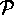, constituting both physical quantities (such as masses of binary components, their radii, luminosities, orbital period, etc., independently of direct observational measurability of these values), and related ``logical'' statements (such as whether the system is binary or not, whether the NS is a radio- or X-ray pulsar or not, whether the radiation from the NS is observable or fully absorbed by a stellar wind, and so on). Each binary traces a path (which we will call a ``track'') in this space, in a way strictly determined by the evolutionary scenario. Each such track emerges with a certain probability, which can be expressed through the initial distribution functions of binary systems, in other terms, through the probability for a binary to be formed with given initial parameters and age. Thus, one can introduce a probability density p in the parameter space.

The most typical questions to be answered with the help of our Scenario Machine are, among others:

1. what is the number or fraction of objects (binaries) lying inside a certain volumeof the space?
2. what is the mean value of a specific parameter x for the binaries from?
3. how do the results depend on the scenario parameters and initial distributions?
4. what type of binary system was a progenitor of the binary with given parameters (i.e. lying within a small volume of the space), and what kind of system can result from it?

Clearly, the first two questions lead to the computation of some integrals over the volume. One possible method which is convenient enough for this task is the Monte-Carlo method.Ā To determine what fraction of binaries of a modeled galaxy will be found in volumeof the parameter space, we must calculate the ratio of the timethe binary remains inside, averaged over all tracks, to the mean duration of all tracks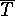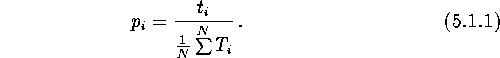For this task to be fulfilled accurately, a large enough number N of evolutionary tracks within a wide range of the initial parameters distributed in accordance with given functions of probability distribution needs to be calculated, and the following quantities must be specified:

(a)
the total timethe tracks stay inside;
(b)
the total duration of all tracks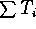required to calculate the average duration of the tracks;
(c)
the sum of squares of durationsrequired to evaluate the accuracy of calculations (using variance D).

Then the fraction of binaries lying insideis expressed by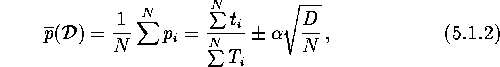whereis a coefficient defined by a confidence level, and the dispersion D is defined as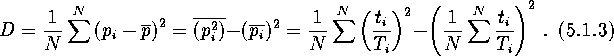To obtain the absolute number of specified binary types, one needs to multiply the quantityby the ratio of the total number of stars in the modeled galaxy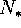to the actual number of modeled tracks N: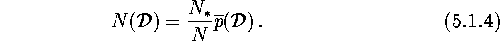To obtain the mean value of some parameter x for the binaries from the volumeof the parameter spacefor N modeled tracks, one needs to calculate quantitiesand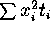. Then the mean value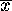is:where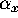is a confidence level, and the variance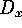is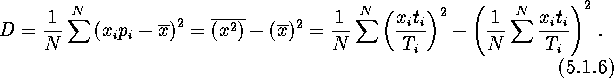Note that all expressions written above can be obtained within the framework of a standard Monte-Carlo methodĀ (see, e.g., Sobol' 1973).Next: Dependence on the Scenario Up: The Scenario Machine: Operational Previous: The Scenario Machine: Operational

Mike E. Prokhorov
Sat Feb 22 18:38:13 MSK 1997